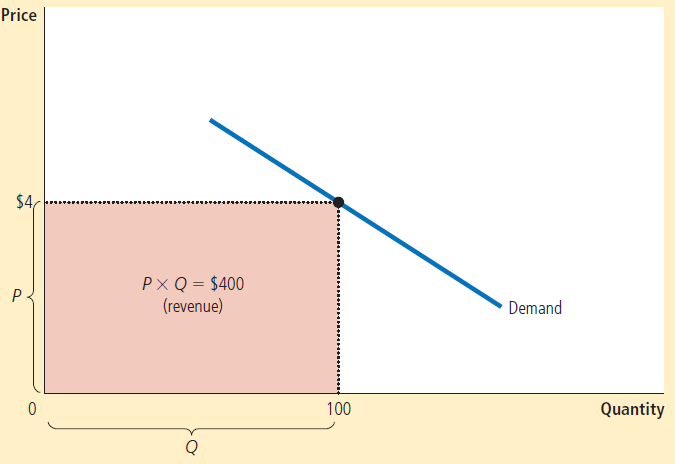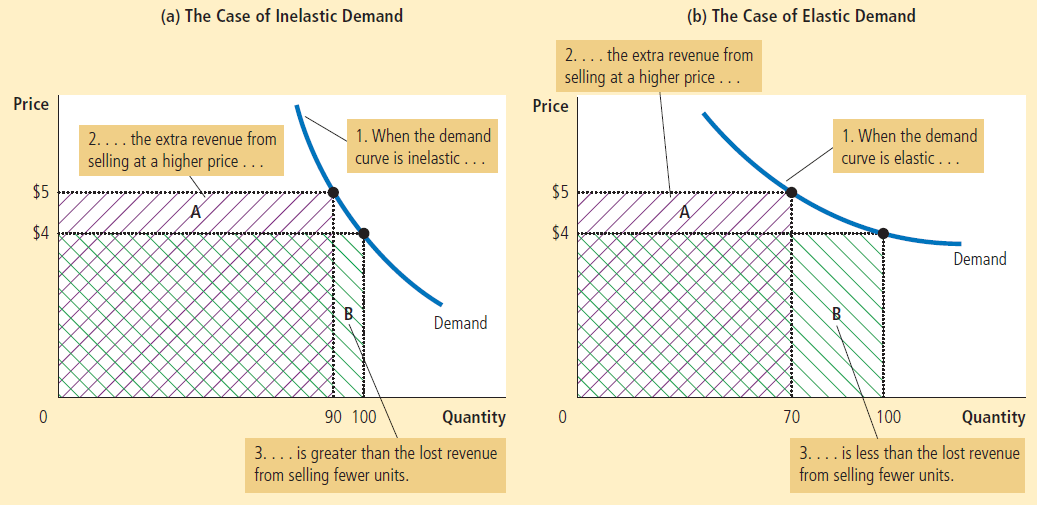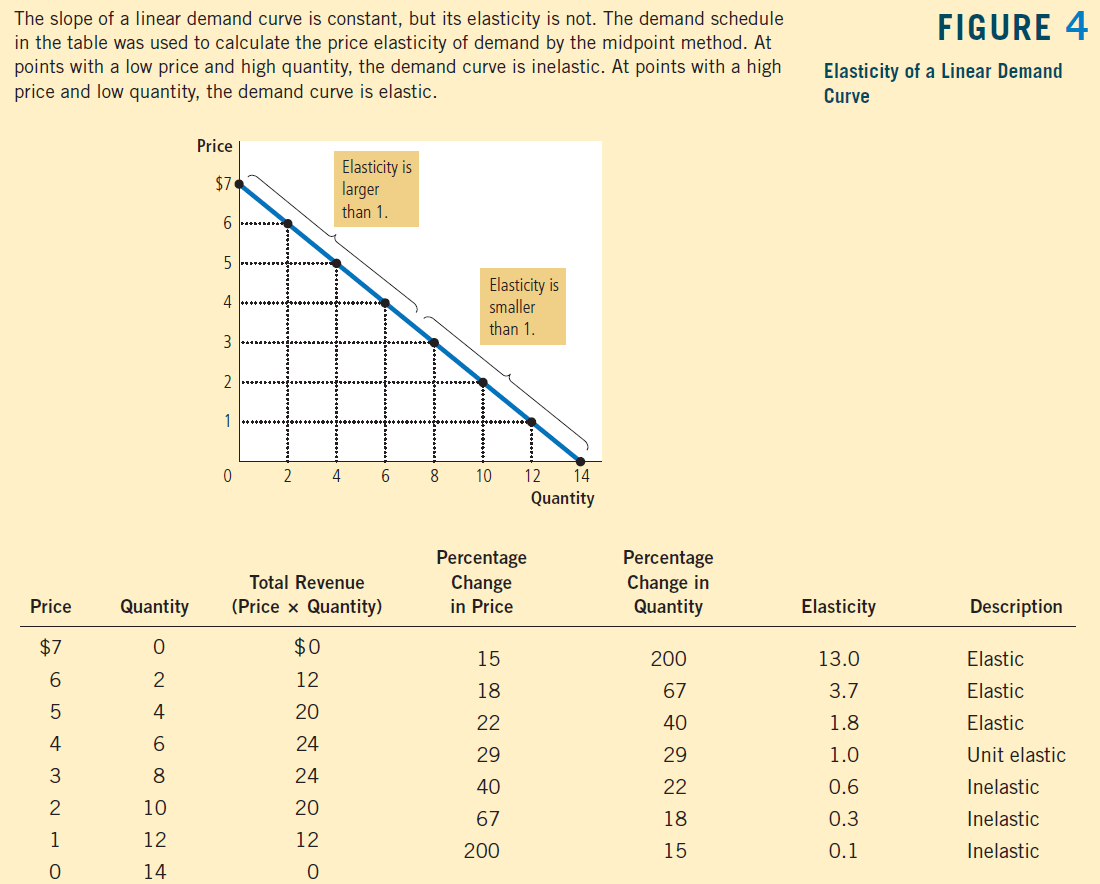# What is Elasticity of Demand

• Price elasticity of demand (PED or Ed)

• Measure used to show the responsiveness, or elasticity, of the quantity demanded of a good or service to a change in price.

• Devised by Alfred Marshall, using the ceteris paribus (all other things being equal) assumption, price elasticity shows by how much quantity changes as a result of a change in price. (Disregard the negative)

• Formula• How to remember

• Queen is greater than the Princess

# The Variety of Demand Curves# Elastic Demand

• When e > 1, demand is elastic, or the percent change in quantity is greater than the percent change in price.

• It means that the product is relatively price-sensitive

• ie. fast-food restaurants, fruits, haircuts

• Demand curve is relatively flat.

# Inelastic Demand

• When e < 1, demand is inelastic, or the percent change in quantity is less than the percent change in price.

• It means that the product is not very sensitive to a change in price

• ie. gasoline, insulin

• Demand curve is relatively steep.

# Price Elasticity of Demand and Total Revenue

• The total amount paid by buyers, and received as revenue by sellers, equals the area of the box under the demand curve.• When demand is inelastic (e < 1), price and total revenue move in the same direction:

• If the price increases, total revenue also increases.
• When demand is elastic (e > 1), price and total revenue move in opposite directions:

• If the price increase, total revenue decreases.
• If demand is unit elastic (e = 1), total revenue remains constant when the price changes.• Examples# Midpioint Method to Find Elasticity

• Definition• Comparison# Factors That Determine Price Elasticity

• Whether close substitutes are available

• Tends to be high if consumers are willing to replace with substitutes.

• Tends to be low if there are no close substitutes

• Whether the good is necessity or a luxury

• Life-saving medication will be inelastic but things you can live without tend to be elastic
• Time

• PED tends to increase over time

• ie. demand for gas is more elastic as behavior changes

• Share of income spend on the good

• Elasticity of demand tends to be low when prices are lower

• Conversely, PED is higher when prices are higher

# Price Elasticity Along the Demand Curve

• Price and total revenue
Inelastic Price↑ Total Revenue↑
Inelastic Price↓ Total Revenue↓
elastic Price↑ Total Revenue↓
elastic Price↓ Total Revenue↑
• Graph• Example